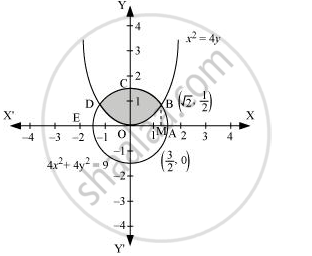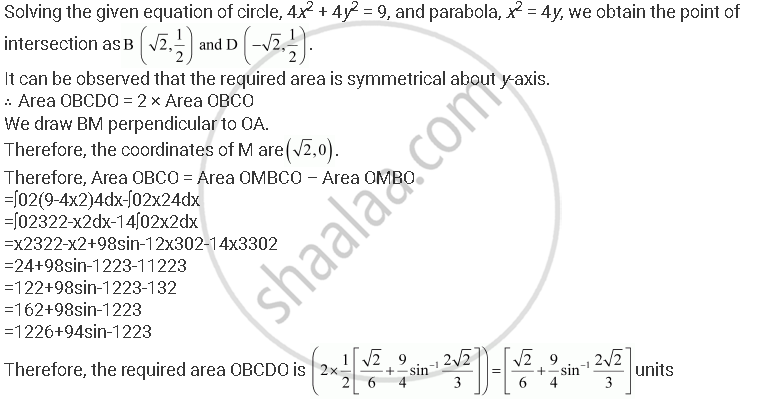Share

# Find the Area of the Circle 4x2 + 4y2 = 9 Which is Interior to the Parabola X2 = 4y - CBSE (Commerce) Class 12 - Mathematics

#### Question

Find the area of the circle 4x2 + 4y2 = 9 which is interior to the parabola x2 = 4y

#### Solution

The required area is represented by the shaded area OBCDO.Is there an error in this question or solution?

#### APPEARS IN

NCERT Solution for Mathematics Textbook for Class 12 (2018 to Current)
Chapter 8: Application of Integrals
Q: 1 | Page no. 371

#### Video TutorialsVIEW ALL 

Solution Find the Area of the Circle 4x2 + 4y2 = 9 Which is Interior to the Parabola X2 = 4y Concept: Area Between Two Curves.
S